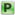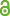Hauptmenü
• Autor
• Berkes, István
• Fukuyama, Katusi
• Nishimura, Takuya
• TitelA Metric Discrepancy Result With Given Speed
• Datei
• DOI10.1007/s10474-016-0658-2
• Persistent Identifier
• Erschienen inActa Mathematica Hungarica
• Band151
• Erscheinungsjahr2017
• Heft1
• Seiten199-216
• LicenceCC BY
• ISSN0236-5294
• Zugriffsrechte• AbstractIt is known that the discrepancy $$D_N\{kx\}$$ quence $$\{kx\}$$ satisfies $$ND_N \{kx\} = O (log N )(log log N )^ {1+ε}$$ a.e. for all $$ε > 0$$, but not for $$ε = 0$$. For $$n_k = θ^k , θ > 1$$ we have $$ND_N \{n_kx\} ≤ (Σ_{θ + ε})(2N log log N )^{1/2}$$ a.e. for some $$0 < Σ_θ < ∞$$ and $$N ≥ N_0 if ε > 0$$, but not for $$ε < 0$$. In this paper we prove, extending results of Aistleitner-Larcher , that for any sufficiently smooth intermediate speed $$Ψ(N)$$ between $$(log N)(log log N)^{1+ε}$$ and $$(N log log N )^{1/2}$$ and for any $$Σ > 0$$, there exists a sequence $$\{n_k\}$$ of positive integers such that $$N D_N \{n_k x\} ≤ (Σ + ε)Ψ(N)$$ eventually holds a.e. for $$ε > 0$$, but not for $$ε < 0$$. We also consider a similar problem on the growth of trigonometric sums.